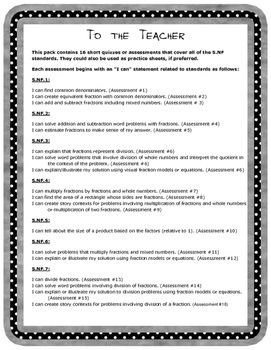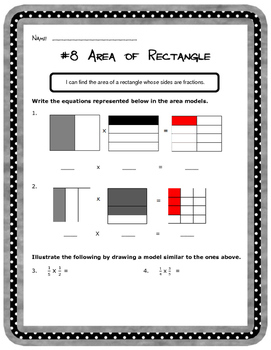# Assessments: All Things Fractions: CCSS 5.NF.1-7Subject
Resource Type
File Type
PDF (717 KB|41 pages)
\$9.00
Product Description

This packet contains 16 short assessments on fractions that cover all of the CCSS for 5.NF.1-7. The assessments could be used as practice sheets or in math centers, if preferred. Each assessment begins with an "I can" statement related to standards. Below is a list of the assessments by standard. The packet is complete with answer keys.

5.NF.1:

I can find common denominators. (Assessment #1)

I can create equivalent fraction with common denominators. (Assessment #2)

I can add and subtract fractions including mixed numbers. (Assessment # 3)

5.NF.2:

I can solve addition and subtraction word problems with fractions. (Assessment #4)

I can estimate fractions to make sense of my answer. (Assessment #5)

5.NF.3:

I can explain that fractions represent division. (Assessment #6)

I can solve word problems that involve division of whole numbers and interpret the quotient in the context of the problem. (Assessment #6)

I can explain/illustrate my solution using visual fraction models or equations. (Assessment #6)

5.NF.4:

I can multiply fractions by fractions and whole numbers. (Assessment #7)

I can find the area of a rectangle whose sides are fractions. (Assessment #8)

I can create story contexts for problems involving multiplication of fractions and whole numbers or multiplication of two fractions. (Assessment #9)

5.NF.5:

I can tell about the size of a product based on the factors (relative to 1). (Assessment #10)

5.NF.6:

I can solve problems that multiply fractions and mixed numbers. (Assessment #11)

I can explain or illustrate my solution using fraction models or equations. (Assessment #12)

5.NF.7:

I can divide fractions. (Assessment #13)

I can solve word problems involving division of fractions. (Assessment #14)

I can explain or illustrate my solution to division problems using fraction models or equations. (Assessment #15)

I can create story contexts for problems involving division of a fraction. (Assessment #16)

Visit Suzanne's Classroom Store!

The resources are designed to enrich or supplement your current curriculum.

Looking for other fraction-related products? Click the links below:

Fraction Quick Tips

Fractions Game Board: Multiplying and Dividing Fractions & Mixed Numbers

Assessments: All Things Fractions (5.NF.1-7)

Fractions Review Board Game: Adding and Subtracting Fractions and Mixed Numbers

Dividing Fractions and Mixed Numbers: 6.NS.A.1

Total Pages
41 pages